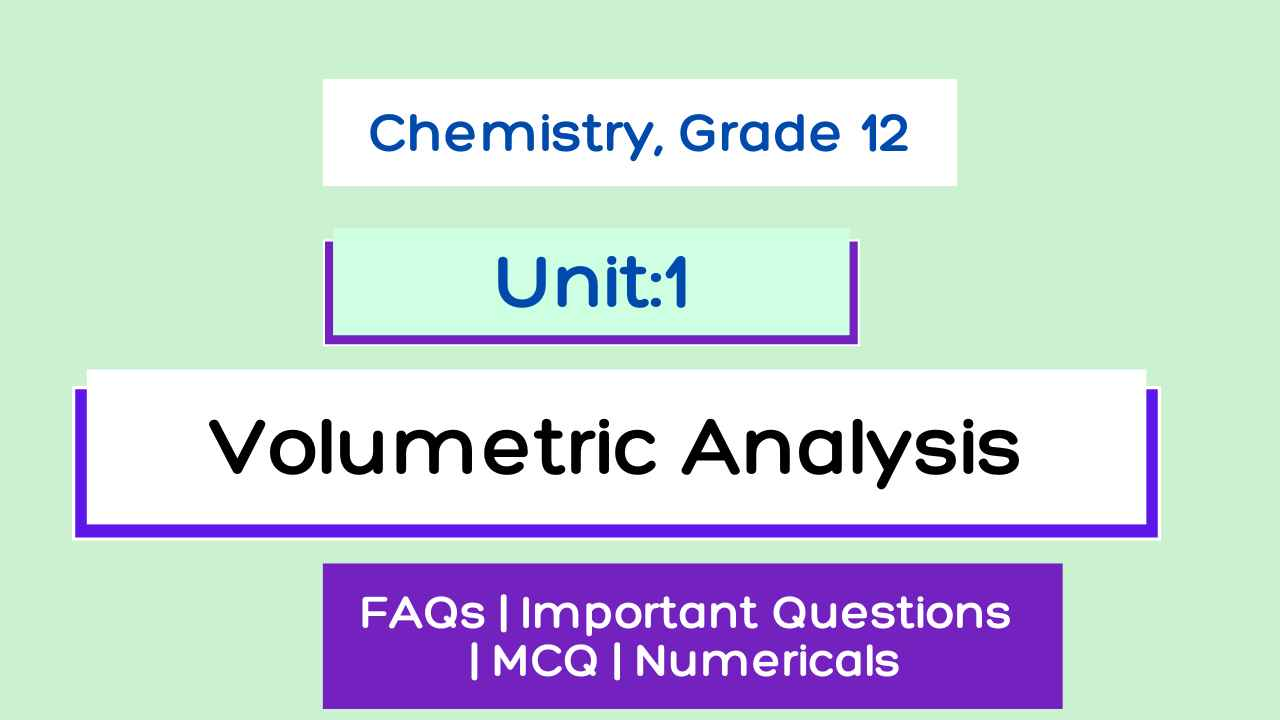# Volumetric Analysis: Class 12 Chemistry Notes

Unit 1: Volumetric Analysis Class 12 Chemistry Notes including Numerical, Important Question for NEB 2080.

Unit 1: Volumetric Analysis

Complete NEB Chemistry 2080 Exercise, Question Answers, MCQs, Numerical, Important Question Note of Class 12 Chemistry Unit 1, Volumetric Analysis.

Unit 2: Ionic Equilibrium Class 12 Chemistry Complete Notes

Volumetric analysis Course content
1.1 Define and explain the terms volumetric and gravimetric analysis.
1.2 Express the concentration of solutions in terms of percentage, g/l, molarity, molality, normality, ppm, ppb
1.3 Define and calculate the equivalent weight of (elements, acids, bases, salts, oxidizing and reducing agents).
1.4 Express the concentration of the solution in terms of normality.
1.5 Explain and apply the concept of the law of equivalence in chemical calculation.
1.6 Define and explain primary and secondary standard substances.
1.7 Explain different types of titration and their applications.Unit 1: Volumetric Analysis

## Volumetric analysis Introduction

Volumetric analysis is defined as the process of the determination of the concentration of an unknown solution by finding its volume which will exactly react with a definite volume of another solution of known concentration.

Gravimetric analysis is defined as the process of quantitative determination of an analyte(the ion being analyzed) based on the mass of a solid.

At first solution of given sample is prepared, treated with suitable reagent to convert into insoluble form which is made pure by washing, dried and weighed. Then the weight of the required substance is calculated with the help of theoretical concept.

Eg. How much SO4-- ion is present in a given sample of Na2SO4?

A known amount of the given sample is dissolved in water and the excess amount of BaCl2 is added to the solution so that all SO4-- is converted into insoluble BaSO4. The precipitate is washed and made pure, it is then dried and weighed. Let the weight of BaSO4 formed is Y gm.

We know, 233 gm. of BaSO4 contains 96 gm. of SO4-- ions.

So, Y gm. of BaSO4 contains (96/233) x Y gm. of SO4--ions.

Pre-requirements in volumetric analysis

Equivalent weight (equivalent mass)

It is defined as “the no. of parts by wt. of the substance that combines with or displaces 1.008 parts by wt. of hydrogen, 8 parts by wt. of oxygen or 35.5 parts by wt. of chlorine.”

Equivalent weight is a unit less.

Equivalent weight expressed in gm is called gm. equivalent.

No. of gm. eqvt. = given wt./eq.wt.

## Volumetric Analysis Complete Notes - NEB Class 12 Chemistry

• Volumetric analysis
• Gravimetric analysis

Eg. How much SO4 -- ion is present in a given sample of Na2SO4?

### Pre-requirements in volumetric analysis

•           Equivalent weight (equivalent mass)

1. Primary standard solution:
2. Secondary standard solution:

#### Normality factor:

Preparation of 250 ml. standard decinormal solution of Na2CO3

Preparation of 250 ml standard decinormal solution of oxalic acid

## Principles of volumetric analysis

### Derivation of normality equation

Que: N1V1 = N2V2 is always true but M1V1 = M2V2 is not true always. Why?

Ans:

The product of normality and volume in litre (NV) gives the no. of g. eq. of the substance. In any reaction, no. of g. eq. of the reacting substances are always equal. Thus, N1V1 = N2V2 is always. The product of molarity and volume in litre (MV) gives the no. of moles of the substance. In any reaction, no. of moles of the reacting substances may or may not be equal. Thus, M,V1 = M2V2 may or may not be true. If no. of moles of reacting substances are equal, M1V1 = M2V2 and If no. of moles of reacting substances are not equal,, M1V1≠M2V2. eg.

2NaOH + H2SO4 → Na2SO4 + 2H2O
80 g.           98 g.            142 g.     36 g.
2 g.eq.        2 mol.
2 g.eq.       1 mol

In the above reaction, the no of g. eq. of NaOH and H2SO, are equal, so N1V1 = N2V2 but the no. of moles are not equal, so M1V1≠M2V2. But in the reaction,

NaOH + HCL → NaCl + H2O
40 g.        36.5 g.
1 g. eq.     1 mol.
1 g. eq.    1 mol

In the above reaction, the no of g. eq. of NaOH and HCl, are equal, so N1V1 = N2V2 and the no. of moles are also equal, so M1V1=M2V2.

## Important apparatus used in the titration.

### Some important terms in volumetric analysis :

• Titration
• Unknown Solution
• Acidimetry
• Alkalimetry
• Titrant
• Titrand
• Indicator
• End Point
• Equivalence Point
• Titration error

## Volumetric Analysis Notes Pdf

This is a Pdf with volumetric analysis notes.

Queries Solved in this Volumetric Analysis Notes:

• Volumetric Analysis Class 12 Notes
• Volumetric Analysis Notes Pdf
• Volumetric Analysis Class 12 Important Questions
• Volumetric Analysis Numerical
• Class 12 Chemistry
• Volumetric analysis Class 12 Exercise Solutions

Getting Info...